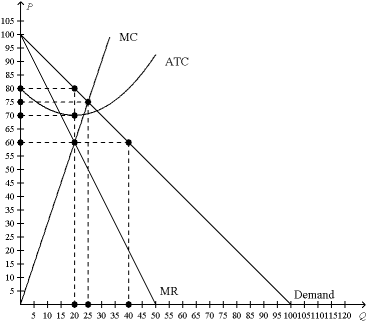/
/
/
Figure 16-2 This figure depicts a situation in a monopolistically competitive
Not my Question
Flag Content

# Question : Figure 16-2 This figure depicts a situation in a monopolistically competitive

Figure 16-2

This figure depicts a situation in a monopolistically competitive market.56. Refer to Figure 16-2. How much profit will the monopolistically competitive firm earn in this situation?

 a. a \$10 profit b. a \$200 profit c. a \$400 profit d. No profit, since monopolistically competitive firms never earn economic profit.

57. Refer to Figure 16-2. How much output will the monopolistically competitive firm produce in this situation?

 a. 20 units b. 25 units c. 40 units d. 80 units

Figure 16-358. Refer to Figure 16-3. The firm in this figure is monopolistically competitive. It illustrates

 a. the shut-down case. b. a long-run economic profit. c. a short-run economic profit. d. a short-run loss.

59. Refer to Figure 16-3. At the profit-maximizing, or loss-minimizing, output level, the firm in this figure has total costs of approximately

 a. \$2,000. b. \$3,000. c. \$4,000. d. \$5,000.

60. Refer to Figure 16-3. Assume the firm in the figure is currently producing 8 units of output and charging \$400. The firm

 a. will increase its profits if it raises its price and reduces its production level. b. will increase its profits if it lowers its price and expands its production level. c. is maximizing profits. d. will increase its profits if it raises its prices and expands its production level.

## Solution 5 (1 Ratings )

Solved
Economics 3 Days Ago 4 Views